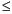Functions and CALL Routines

# RANNOR Function

Returns a random variate from a normal distribution.
 Category: Random Number Tip: If you want to change the seed value during execution, you must use the CALL RANNOR routine instead of the RANNOR function.

## Syntax

 RANNOR(seed)

### Arguments

seed

is a numeric constant, variable, or expression with an integer value. If seed0, the time of day is used to initialize the seed stream.

The RANNOR function returns a variate that is generated from a normal distribution with mean 0 and variance 1. The Box-Muller transformation of RANUNI uniform variates is used.

A normal variate X with mean MU and variance S2 can be generated with this code:

`x=MU+sqrt(S2)*rannor(seed);`

A lognormal variate X with mean exp(MU + S2/2) and variance exp(2*MU + 2*S2) -exp(2*MU + S2) can be generated with this code:

`x=exp(MU+sqrt(S2)*rannor(seed));`

For a discussion about seeds and streams of data, as well as examples of using the random-number functions, see Generating Multiple Variables from One Seed in Random-Number Functions.

The CALL RANNOR routine, an alternative to the RANNOR function, gives greater control of the seed and random number streams.

 Functions and CALL routines:Previous Page | Next Page | Top of Page